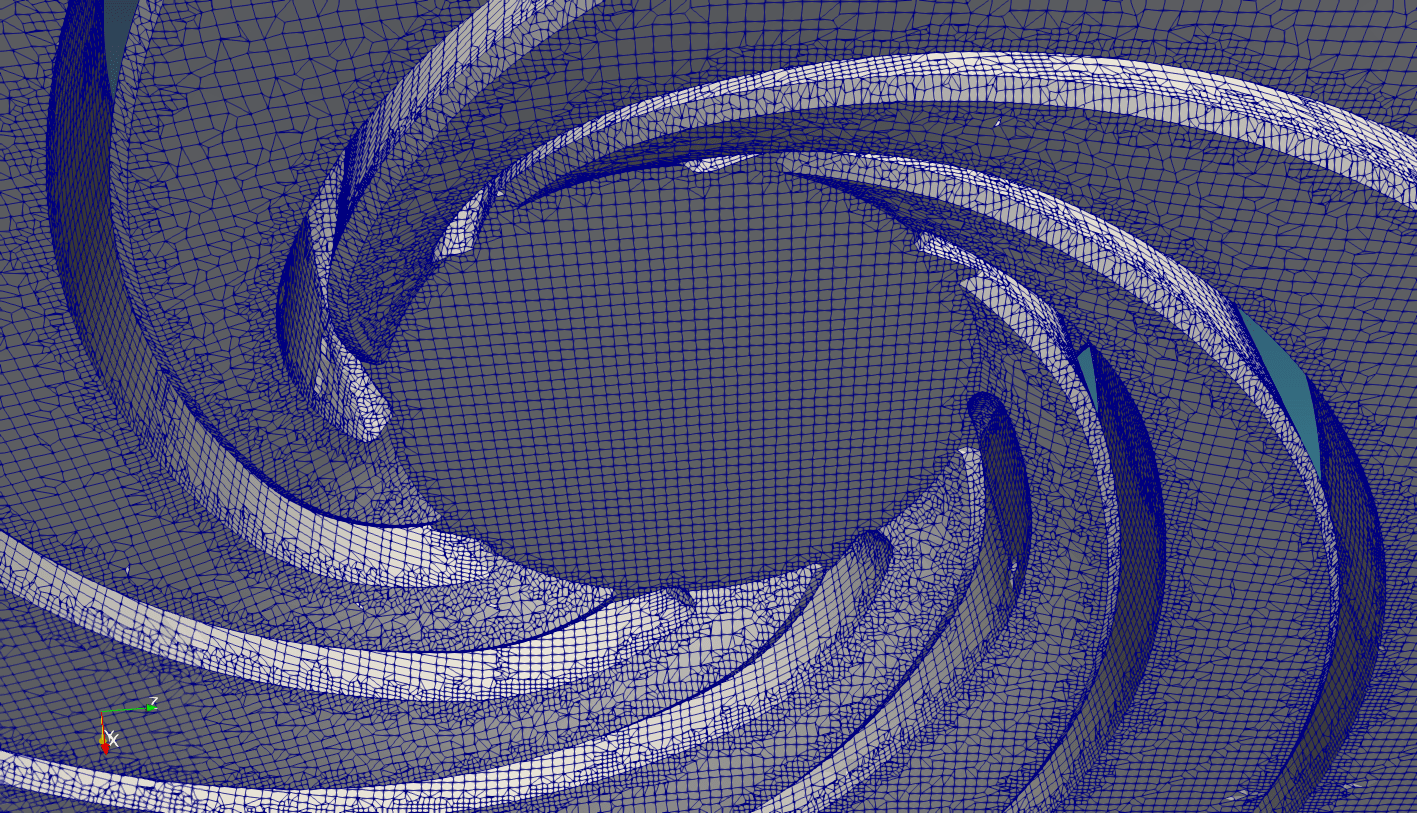# OpenFOAM meshing: a few shortcuts

## Merging Files

If you’re using SALOME to create STL files for meshing you can only save separate surfaces to separate files. That’s not a problem if you’re using snappyHexMesh but cfMesh only takes an STL file that has all surfaces combined and named. I wrote a simple Python script that does that for you. Save STL files as ASCII, change the names in the first few lines and run the script.

# -*- coding: utf-8 -*-

# the directory of your STL files
directory = "../geometry/impeller/"

# STL files that will be merged into one
input_names = [
"impeller_walls",
"impeller_inlet",
"impeller_outlet",
]

# name of the output STL file
output_name = "impeller"

############################
############################
############################
extension = ".stl"

output_file = open(output_name + extension, "w")

for name in input_names:
f = open(directory + name + extension, "r")

output_file.write("solid " + name + "\n")

while line != "":
output_file.write(line)

f.close()

output_file.close()


## Background mesh

If you’re using snappyHexMesh you can input separate STL file but need a background mesh. I grew tired of manually updating blockMeshDict every time I update geometry so I wrote another Python script that does that for me.

It takes a merged STL file as input (I often mesh with both snappyHexMesh and cfMesh and the decide which one works better so the above script should be run first) and then finds the minimum and maximum values for vertices in all dimensions and writes a blockMeshDict file.

Note that this is only useful for meshing inside STL surfaces, not outside. Also remember to update locationInMesh in snappyHexMeshDict if your geometry changed drastically.

You don’t need to update this script because it takes command-line arguments:

• File: ASCII STL file that will be used for meshing
• Hex size: size of hexahedra in background mesh (will always be slightly less)
• Expand factor: optional; the background mesh should not coincide with any of the surfaces in STL. So it is made 0.1% bigger by default but you can specify your own value.

This is the script:

import re
import math
import sys
import os

# command-line input
help_text = """Usage: makeBMD.py <file> <hex size [m]> [expand_factor] """

try:
if len(sys.argv) < 3:
raise ValueError

file_name = sys.argv
cell_size = float(sys.argv)

if len(sys.argv) > 3:
expand_factor = float(sys.argv)
else:
expand_factor = 1.001
except:
print sys.argv
print help_text
sys.exit()

# format of vertex files:
#    vertex  7.758358e-03  2.144992e-02  1.539336e-02
#    vertex  7.761989e-03  2.167315e-02  1.525611e-02
#    vertex  7.767175e-03  2.167225e-02  1.551236e-02

# a regular expression to match a beginning of a vertex line in STL file
vertex_re = re.compile('\s+vertex.+')

vertex_max = [-1e+12, -1e+12, -1e+12]
vertex_min = [ 1e+12,  1e+12,  1e+12]

# stroll through the file and find points with highest/lowest coordinates
with open(sys.argv, 'r') as f:
for line in f:
m = vertex_re.match(line)

if m:
n = line.split()
v = [float(n[i]) for i in range(1, 4)]

vertex_max = [max([vertex_max[i], v[i]]) for i in range(3)]
vertex_min = [min([vertex_min[i], v[i]]) for i in range(3)]

# scale the blockmesh by a small factor
# achtung, scale around object center, not coordinate origin!
for i in range(3):
center = (vertex_max[i] + vertex_min[i])/2
size = vertex_max[i] - vertex_min[i]

vertex_max[i] = center + size/2*expand_factor
vertex_min[i] = center - size/2*expand_factor

# find out number of elements that will produce desired cell size
sizes = [vertex_max[i] - vertex_min[i] for i in range(3)]
num_elements = [int(math.ceil(sizes[i]/cell_size)) for i in range(3)]

print "max: {}".format(vertex_max)
print "min: {}".format(vertex_min)
print "sizes: {}".format(sizes)
print "number of elements: {}".format(num_elements)
print "expand factor: {}".format(expand_factor)

# write a blockMeshDict file
bm_file = """
/*--------------------------------*- C++ -*----------------------------------*\
| =========                 |                                                 |
| \\\\      /  F ield         | OpenFOAM: The Open Source CFD Toolbox           |
|  \\\\    /   O peration     | Version:  dev                                   |
|   \\\\  /    A nd           | Web:      www.OpenFOAM.org                      |
|    \\\\/     M anipulation  |                                                 |
\*---------------------------------------------------------------------------*/
FoamFile
{{
version     2.0;
format      ascii;
class       dictionary;
object      blockMeshDict;
}}
// * * * * * * * * * * * * * * * * * * * * * * * * * * * * * * * * * * * * * //

convertToMeters 1;

x_min {v_min};
x_max {v_max};

y_min {v_min};
y_max {v_max};

z_min {v_min};
z_max {v_max};

n_x {n};
n_y {n};
n_z {n};

vertices
(
($x_min$y_min $z_min) //0 ($x_max $y_min$z_min) //1
($x_max$y_max $z_min) //2 ($x_min $y_max$z_min) //3
($x_min$y_min $z_max) //4 ($x_max $y_min$z_max) //5
($x_max$y_max $z_max) //6 ($x_min $y_max$z_max) //7
);

blocks ( hex (0 1 2 3 4 5 6 7) ($n_x$n_y \$n_z) simpleGrading (1 1 1) );

edges ( );
patches ( );
mergePatchPairs ( );

// ************************************************************************* //
"""

with open(os.path.join('system', 'blockMeshDict'), 'w') as mesh_file:
mesh_file.write(
bm_file.format(v_min=vertex_min, v_max=vertex_max, n=num_elements)
)

print "done."



## SnappyHexMesh: my procedureNote that I always mesh in separate directories / cases and then copy constant/polyMesh to the actual simulation case. controlDict in meshing case always starts from 0 and deltaT is 1 so that snappyHexMesh always produces directories 1 2 3 for castellation, snapping and layer addition. If I was running a pimpleFoam case, for example, snappy would produce 1e-6, 2e-6 and 3e-6 if my deltaT was 1e-6. Which is not very handy.

This is a script I use for meshing with snappyHexMesh:

# prepare your STL files and edit this script to match their names and directories
python joinstl.py

mv impeller.stl constant/triSurface

# create a background mesh from the prepared model
python makeBMD.py constant/triSurface/impeller.stl 0.001 1.001

# remove relics from old tries
rm -r 1 2 3

# edges and other features; system/surfaceFeatureExtractDict must be defined
surfaceFeatureExtract

# background mesh
blockMesh

# meshing in parallel: system/decomposeParDict must be defined;
# use mpiexec on windows and mpirun on linux
decomposePar -force
mpiexec -np 4 snappyHexMesh -parallel
reconstructParMesh -latestTime

# sets and zones, if there's anything in system/topoSetDict;
# also, updates on patches if there's anything in system/changeDictionaryDict
topoSet
setsToZones
changeDictionary

# check and see
checkMesh
paraFoam


Hope this is of some help. Good luck!